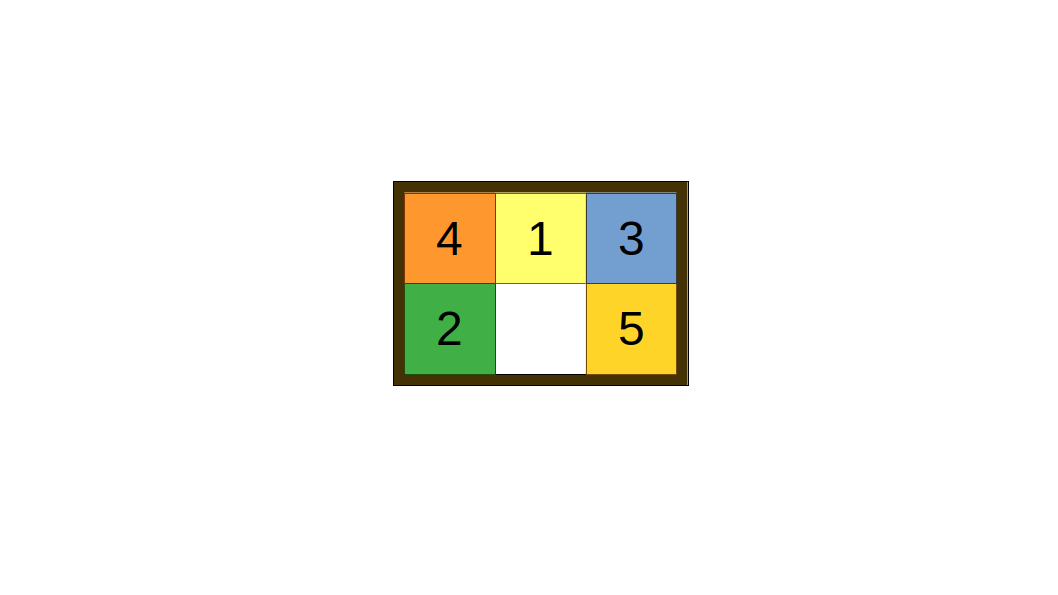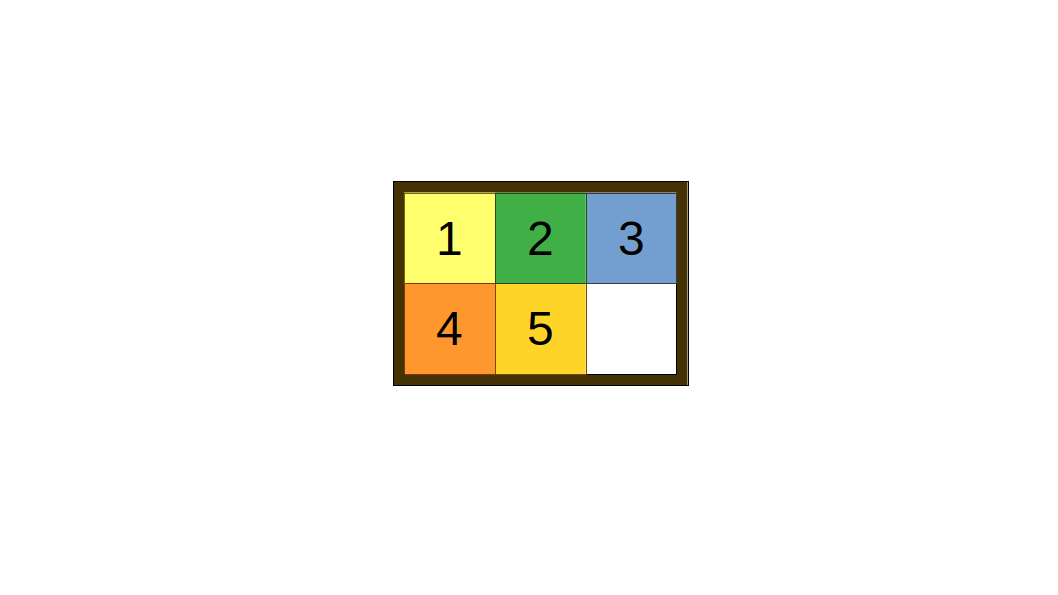# Sliding Puzzle

You happen upon a puzzle box that unlocks... Something. You aren't quite sure what just yet. The puzzle is a `2 x 3` box, and there are `5` square sliding bricks labelled conveniently with `1` to `5`. It looks something like this:It can be represented as a `2 x 3` matrix containing numbers from `0` to `5`, where `0` represents an empty space. For example, the pattern above can be represented by `[[4, 1, 3], [2, 0, 5]]`.

The tiles are interlocked with each other, so you cannot take the tiles out. However, the tiles can move freely horizontally and vertically, so each turn, you can move a tile to an adjacent empty space. You have a feeling that when you move the tiles to the position `[[1, 2, 3], [4, 5, 0]]`, the puzzle will be solved and unlock the "something". Like this:You need to be quick about solving this puzzle, though, as you might attract the attention of someone who doesn't like you poking around in their dungeon. You wonder whether it is possible to solve this puzzle, and if so, how many steps you need to move in order to do so.

### Input

• `init_pos`: an integer matrix representing the initial position of the puzzle.

### Output

The number of steps required to solve this puzzle, or `-1` if the puzzle is impossible to solve.

### Examples

#### Example 1:

Input:

``1init_pos = [[4, 1, 3], [2, 0, 5]]``

Output: `5`

Explanation:

### Constraints

• The input must be a `2 x 3` integer matrix containing exactly one of each from `0` to `5`